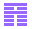# Dynamic Computation

Thore Husfeldt

December 1997

### Abstract:

Chapter 1 contains a brief introduction and motivation of dynamic computations, and illustrates the main computational models used throughout the thesis, the random access machine and the cell probe model introduced by Fredman.

Chapter 2 paves the road to proving lower bounds for several dynamic problems. In particular, the chapter identifies a number of key problems which are hard for dynamic computations, and to which many other dynamic problems can be reduced. The main contribution of this chapter can be summarised in two results. The first shows that the signed prefix sum problem, which has already been heavily exploited for proving lower bounds on dynamic algorithms and data structures, remains hard even when we provide some amount of nondeterminism to the query algorithms. The second result studies the amount of extra information that can be provided to the query algorithm without affecting the lower bound. Some applications of these results are contained in this chapter; in addition, they are heavily developed for the lower bound proofs in the remainder of the thesis.

Chapter 3 investigates the dynamic complexity of the symmetric Boolean functions, and provides upper and lower bounds. These results establish links between parallel complexity (namely, Boolean circuit complexity) and dynamic complexity. In particular, it is shown that the circuit depth of any symmetric function and the dynamic prefix problem for the same function depend on the same combinatorial properties. The connection between these two different modes and models of computation is shown to be very strong in that the trade-off between circuit size and circuit depth is similar to the trade-off between update and query time.

Chapter 4 considers dynamic graph problems. In particular, it presents the fastest known algorithm for dynamic reachability on planar acyclic digraphs with one source and one sink (known as planar st-graphs). Previous partial solutions to this problem were known. In the second part of the chapter, the techniques for lower bound from chapter 2 are further exploited to yield new hardness results for a number of graph problems, including reachability problems in planar graphs and grid graphs, dynamic upward planarity testing and monotone point location.

Chapter 5 turns to strings, and focuses on the problem of maintaining a string of parentheses, known as the dynamic membership problem for the Dyck languages. Parentheses are inserted and removed dynamically, while the algorithm has to check whether the string is properly balanced. It is shown that this problem can be solved in polylogarithmic time per operation. The lower bound techniques from the thesis are again used to prove the hardness of this problem

Available as PostScript, PDF.BRICS WWW home page